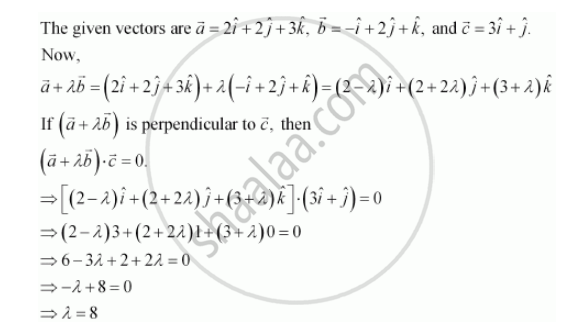Share

# If Veca = 2hati + 2hatj + 3hatk , Vecb = -veci + 2hatj + Hatk and Vecc = 3hati + Hatj Are Such that Veca + Lambdavecb is Perpendicular to Vecc, Then Find the Value Of λ. - CBSE (Science) Class 12 - Mathematics

ConceptProduct of Two Vectors Projection of a Vector on a Line

#### Question

If veca = 2hati + 2hatj + 3hatk , vecb = -veci + 2hatj + hatk and vecc = 3hati + hatj are such that veca + lambdavecb is perpendicular to vecc, then find the value of λ.

#### SolutionHence, the required value of λ is 8.

Is there an error in this question or solution?

#### Video TutorialsVIEW ALL 

Solution If Veca = 2hati + 2hatj + 3hatk , Vecb = -veci + 2hatj + Hatk and Vecc = 3hati + Hatj Are Such that Veca + Lambdavecb is Perpendicular to Vecc, Then Find the Value Of λ. Concept: Product of Two Vectors - Projection of a Vector on a Line.
S